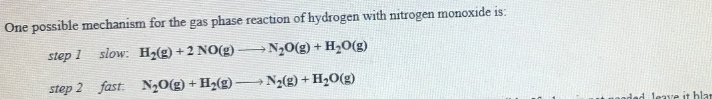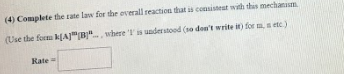# One possible mechanism for the gas phase reaction of hydrogen with nitrogen monoxide is: step 1 slow: H2(g) + 2 NO(g) → N2O(g) + H2O(g) step 2 fast: N2O(g) + H2(g) → N2(g) + H2O(g) (4) Complete the rate law for the overall reaction that is consistent with this mechanism. (use the form k[A]^m[B]^n... , where '1' is understood (so don't write it) for m, n etc.)